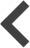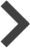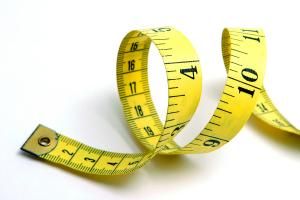20% off all wall art!   Today only!   Offer ends tonight at midnight EST.

# Imperial to metric conversion chart - from inches to centimetres

Helene U Taylor

Blog #2 of 2Previous

|

NextFebruary 10th, 2013 - 08:31 PMIf you are not familiar with ordering prints using imperial measurements, please use the table below for conversion.

Portrait size:
6.00" x 8.00" = 15.2 x 20.3 cm
7.50" x 10.00" = 19.0 x 25.4 cm
9.00" x 12.00" = 22.8 x 30.4 cm
10.50" x 14.00" = 26.6 x 35.5 cm
12.00" x 16.00" = 30.4 x 40.6 cm
15.00" x 20.00" = 38.1 x 50.8 cm
18.00" x 24.00" = 45.7 x 60.9 cm
22.50" x 30.00" = 57.1 x 76.2 cm
27.00" x 36.00" = 68.5 x 91.4 cm
30.00" x 40.00" = 76.2 x 101.6 cm
36.00" x 48.00" = 91.4 x 121.9 cm
45.00" x 60.00" = 114.3 x 152.4 cm

Landscape size:
8.00" x 6.00" = 20.3 x 15.2 cm
10.00" x 7.50" = 25.4 x 19.0 cm
12.00" x 9.00" = 30.4 x 22.8 cm
14.00" x 10.50" = 35.5 x 26.6 cm
16.00" x 12.00" = 40.6 x 30.4 cm
20.00" x 15.00" = 50.8 x 38.1cm
24.00" x 18.00" = 60.9 x 45.7 cm
30.00" x 22.50" = 76.2 x 57.1cm
36.00" x 27.00" = 91.4 x 68.5 cm
40.00" x 30.00" = 101.6 x 76.2 cm
48.00" x 36.00" = 121.9 x 91.4 cm
60.00" x 45.00" = 152.4 x 114.3 cm